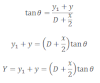# Motion Of Charge Particle In Electric Field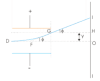Let us consider a charged particle having charge ‘q’ moves with horizontal velocity ‘v’, enters in the region of electric field strength (E) as shown in the fig. As the charged particle moves in the electric field it deviates towards the positive plate. P1. Let y be the vertical distance which the charged particle just emerges from the electric field.
Now, since initial velocity is moving with horizontal component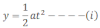Also, according to Newton’s law,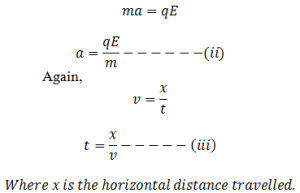Now, from equation (i), (ii) and (iii) we get,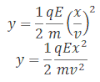This equation shows that the path followed by charged particle is parabolic in nature.

Now, the vertical component of velocity can be obtained as: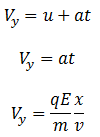Now the net velocity of the charged particle when it just emerges from the electric field is,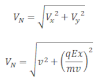Deflection of the charged particle from its initial path can be obtained as: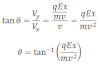Deflection at the screen can be obtained as: